# Simulation Manual: Refraction of Light

## Introduction:

With this simulation, you can experiment the refraction of light between air and a transparent semi-disk.

You can choose the material of the disk from a list of materials.

Also, you can determine the index of refraction of the semi-disk when you apply Snell’s law to the measurements you take in the simulation.## The target users:

This simulation is instrumental and informative for students who want to virtually experiment without the need for a real lab (or in the case of lab equipment shortage).

The simulation is also invaluable and handy for teachers and lab instructors who want to engage their students in performing lab activities on the refraction of light and draw conclusions and discover the underlying principle, which is Snell’s law. Alternatively, they can engage their students in experiments where they apply Snell’s law to determine the index of refraction of a certain transparent substance.

I advise the instructors who want to benefit from this simulation to introduce the experiment in a directed discovery approach. This way, the students are guided through repeating the experiment to discover the law mentioned above rather than receiving it.

## Importance of the simulation:

Optics experiments are intricate physics experiments. They are usually performed in a dark room and require expensive equipment, and require a lot of preparation and precise measurement. This equipment is not easily found in every school lab. So, this simulation makes it easy for high-school and undergraduate students to perform an affordable activity that enables them to acquire the required skills and knowledge in this topic.

Of course, it is much better to perform a real experiment instead of a virtual experiment when possible. However, the virtual experiment is much appreciated in the case of a shortage of equipment or for a preliminary activity to prepare the students for the real lab.

Instructional designers and course creators may find that this simulation facilitates their work.

## A short introduction to the refraction of light and Snell’s law:

Refraction of light is the bending of a light ray as it passes from one transparent medium to another due to the difference in the speeds of light in different media.

Refraction is always accompanied by a partial reflection at the surface separating the two mediums (that is why you can see your image on the surface of a lake).

The following figure defines various elements in the phenomenon of the refraction of light.

### Definitions:

• Surface of separation (or interface): is the surface that separates the two transparent mediums.• Incident ray: is the light ray that hits the surface of separation at one side.
• Refracted ray: is the light ray that emerges to the other side of the surface of separation.
• Point of incidence: is a point on the surface of separation on which the incident ray hits the surface.
• Normal: is a straight line drawn perpendicular to the surface of separation at the point of incidence.
• Angle of incidence: is the angle between the incident ray and the normal.
• Angle of refraction: is the angle between the refracted ray and the normal.

### Index of refraction:

The speed of light differs from one medium to another and has its highest value in vacuum, which is c = 3×108 m/s (The speed of light in air is almost the same, so we can treat air approximately as vacuum in such experiments).

The index of refraction of a medium is denoted by “n” and is defined as:Note that n ≥ 1 since we always have v ≤ c

Note that n is unitless.

Consider two transparent media A and B: if the index of refraction of the medium A (or simply the index of A) is less than the index of B (that is, nA < nB), then we say that:

A is less refractive than B or B is more refractive than A.

### Passage of light from one transparent medium to another:

• First case: normal incidence

In this case, the ray will continue non-deviated: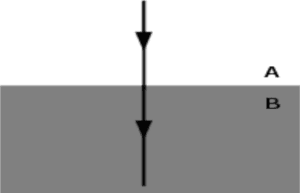• Second case: the passage of light from a medium A to a more refractive medium B (nA < nB)

For example, from air to glass or from air to water.

In this case, the ray will bend towards the normal: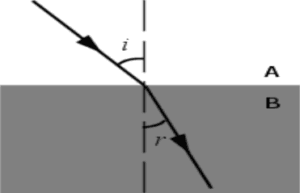• Third case: the passage of light from medium A to a less refractive medium B (nA > nB)

For example, from glass to air or from water to air.

In this case, the ray will bend away from the normal: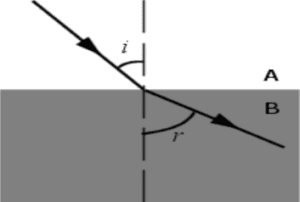### Total internal reflection:

• Total internal reflection may happen only when light is passing from a medium A to a less refractive medium B, that is nA > nB (for example from glass to air or from water to air), and the angle of incidence is greater than a certain value which is called the critical angle, or the limiting angle, and is denoted by λ or iL.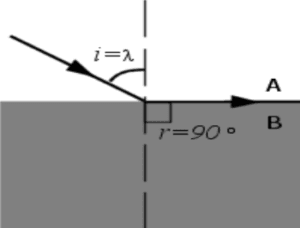• How to determine the critical angle of a medium: the critical angle is the incident angle that corresponds to a refraction angle of 90°, which is called the grazing angle. In this case, the refracted ray emerges grazing the surface of separation.
• Conclusion: when light passes from a transparent medium A to another transparent medium B where nA > nB:

1. if then refraction will take place.

2. if then the refraction angle is 90° (grazing angle).

• if then total internal reflection will take place and  (where  is the angle of reflection and not refraction).

### Snell’s law:

Snell’s law is used in geometric optics to calculate the angle of incidence or the angle of refraction, and in experimental optics to find the refractive index of a material. The formula is:Where i1 is the angle of incidence in medium 1 and i2 is the angle of refraction in medium 2. n1 is the index of refraction of medium 1 and n2 is the index of refraction of medium 2.

## Working guidelines for the simulations:

In this section, we are going through each element of the simulation and explain its function.

Note that you can also go through this tutorial in the simulation by clicking on the “Tutorial” button.1. Maximize / Minimize toggle button: Click this button to enter full-screen mode or to restore the window mode.2. Semi-circular disk: This is made up of a transparent material that you can choose from the “Medium1” menu. Note that Medium 2 is air.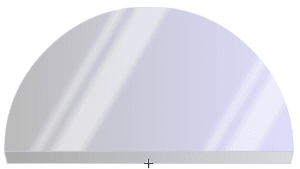3. “Medium1” menu: drop-down this menu to choose among available mediums that the semi-disk is made up of.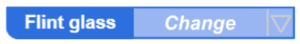4. Protractor: You can choose one of two protractors, one with fewer graduations and another with full graduations for precise measurements.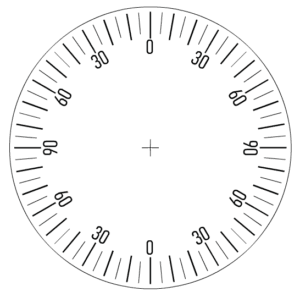5. Show Detailed Protractor: Tick this check-button to use another protractor with full graduations for precise measurements.6. Light Source: This gives a narrow light beam (light ray). Rotate it around the protractor to vary the angle of incidence.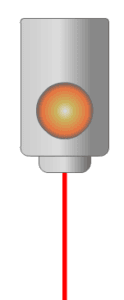7. ON-OFF button: click this button to turn ON or OFF the light source.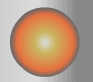## Conclusion:

With this simulation, which is rich with controls and visuals, you can observe the refraction of light and verify Snell’s law, and with the proper instructional methodologies, the instructor will be able to empower the sense of discovery in the learners and introduce the concept of refraction in a clear and visually rich presentation and enable the learners to acquire the required learning objectives.

It is worth mentioning that this simulation is coded with the latest HTML5/JavaScript web tools.